Plot an fcm object as a network, where edges show co-occurrences of features.

textplot_network(
x,
min_freq = 0.5,
omit_isolated = TRUE,
edge_color = "#1F78B4",
edge_alpha = 0.5,
edge_size = 2,
vertex_color = "#4D4D4D",
vertex_size = 2,
vertex_labelcolor = NULL,
vertex_labelfont = NULL,
vertex_labelsize = 5,
offset = NULL,
...
)

# S3 method for fcm
as.network(x, min_freq = 0.5, omit_isolated = TRUE, ...)

# S3 method for fcm
as.igraph(x, min_freq = 0.5, omit_isolated = TRUE, ...)

## Arguments

x a fcm or dfm object a frequency count threshold or proportion for co-occurrence frequencies of features to be included. if TRUE, features do not occur more frequent than min_freq will be omitted. color of edges that connect vertices. opacity of edges ranging from 0 to 1.0. size of edges for most frequent co-occurrence The size of other edges are determined proportionally to the 99th percentile frequency instead of the maximum to reduce the impact of outliers. color of vertices. size of vertices color of texts. Defaults to the same as vertex_color. If NA is given, texts are not rendered. font-family of texts. Use default font if NULL. size of vertex labels in mm. Defaults to size 5. Supports both integer values and vector values. if NULL, the distance between vertices and texts are determined automatically. additional arguments passed to network or graph_from_adjacency_matrix. Not used for as.igraph.

## Details

Currently the size of the network is limited to 1000, because of the computationally intensive nature of network formation for larger matrices. When the fcm is large, users should select features using fcm_select, set the threshold using min_freq, or implement own plotting function using as.network().

fcm()

network::network()

igraph::graph_from_adjacency_matrix()

## Author

Kohei Watanabe and Stefan Müller

## Examples

set.seed(100)
toks <- data_char_ukimmig2010 %>%
tokens(remove_punct = TRUE) %>%
tokens_tolower() %>%
tokens_remove(pattern = stopwords("english"), padding = FALSE)
fcmat <- fcm(toks, context = "window", tri = FALSE)
feat <- names(topfeatures(fcmat, 30))
fcm_select(fcmat, pattern = feat) %>%
textplot_network(min_freq = 0.5)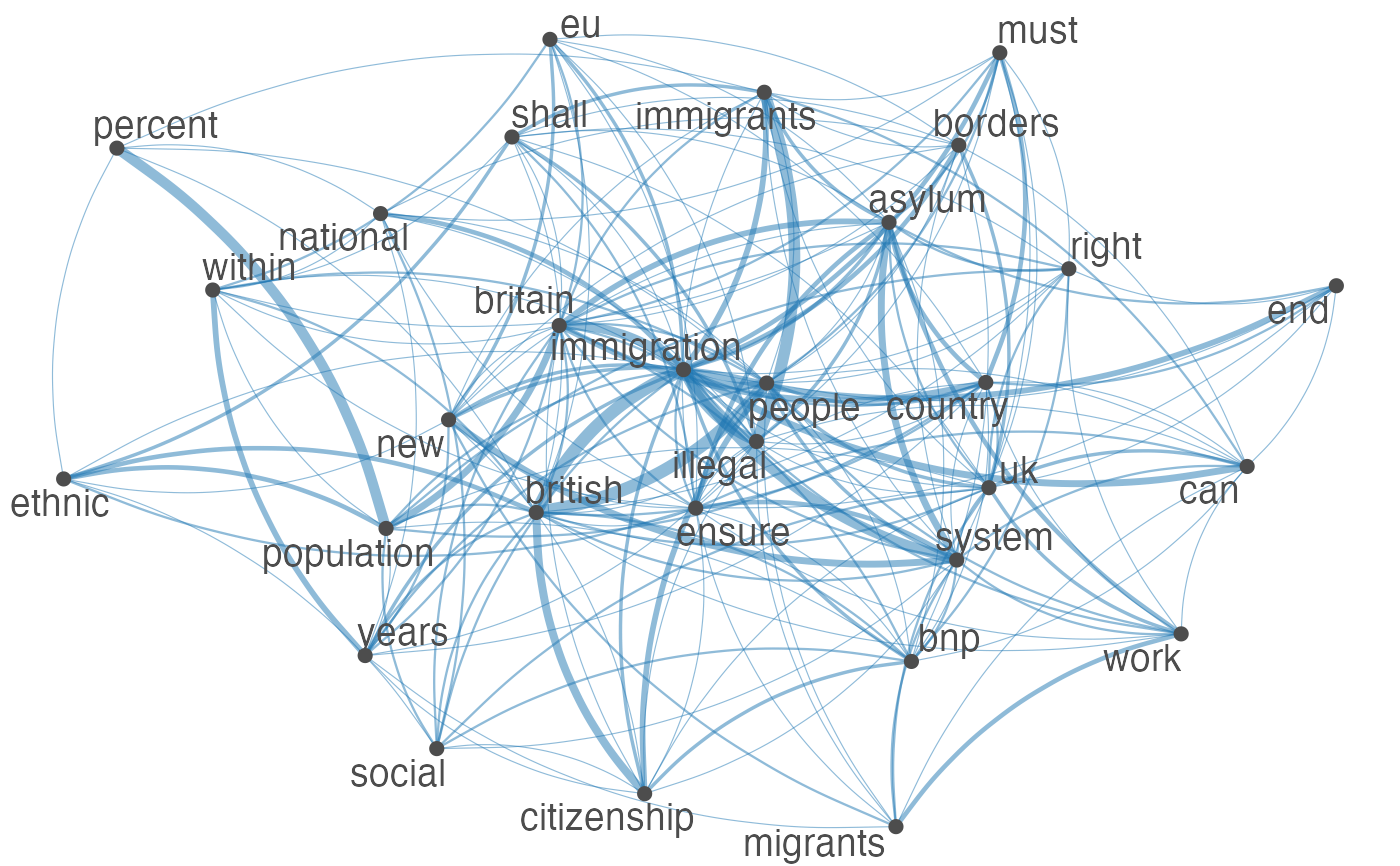fcm_select(fcmat, pattern = feat) %>%
textplot_network(min_freq = 0.8)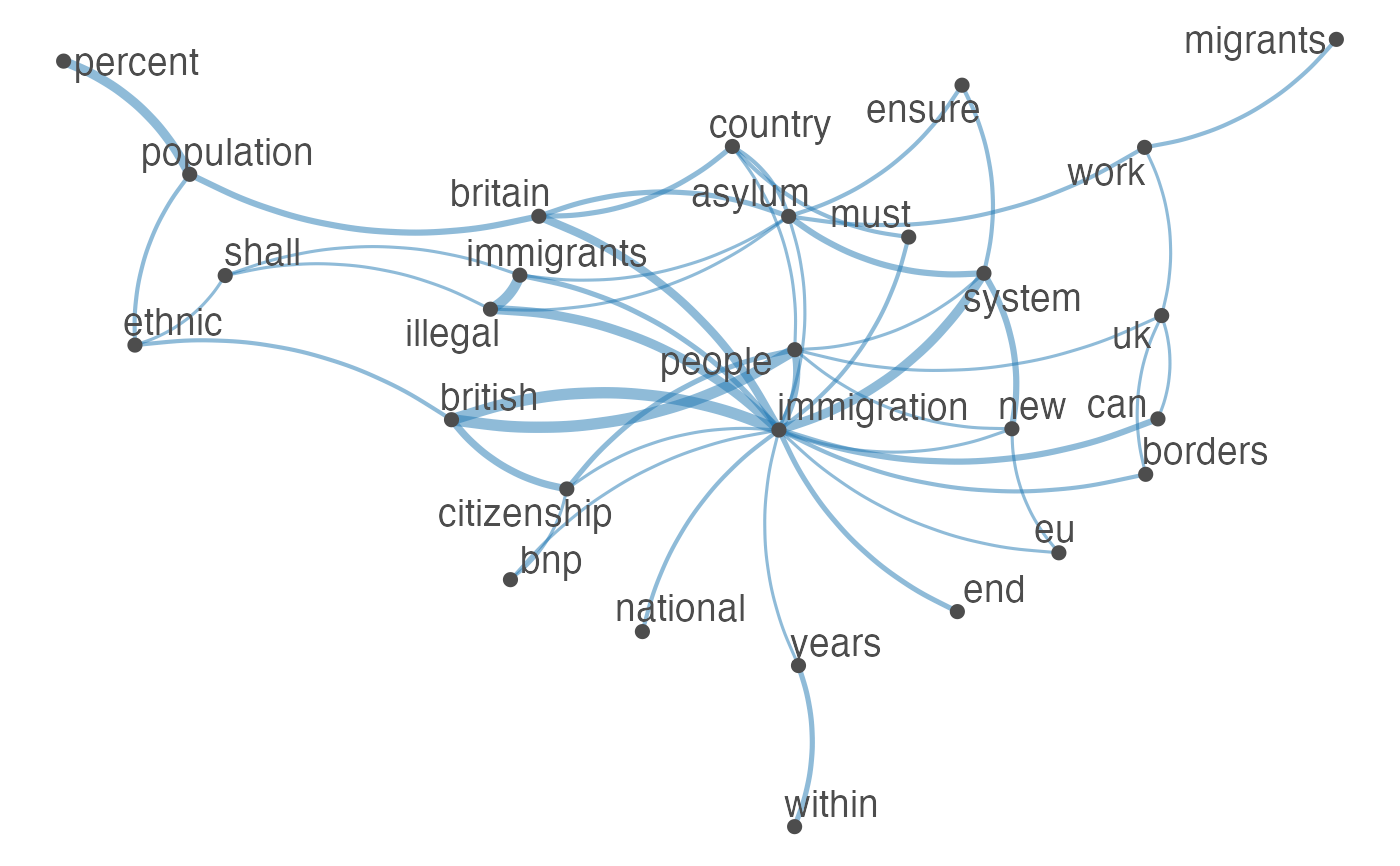fcm_select(fcmat, pattern = feat) %>%
textplot_network(min_freq = 0.8, vertex_labelcolor = rep(c('gray40', NA), 15))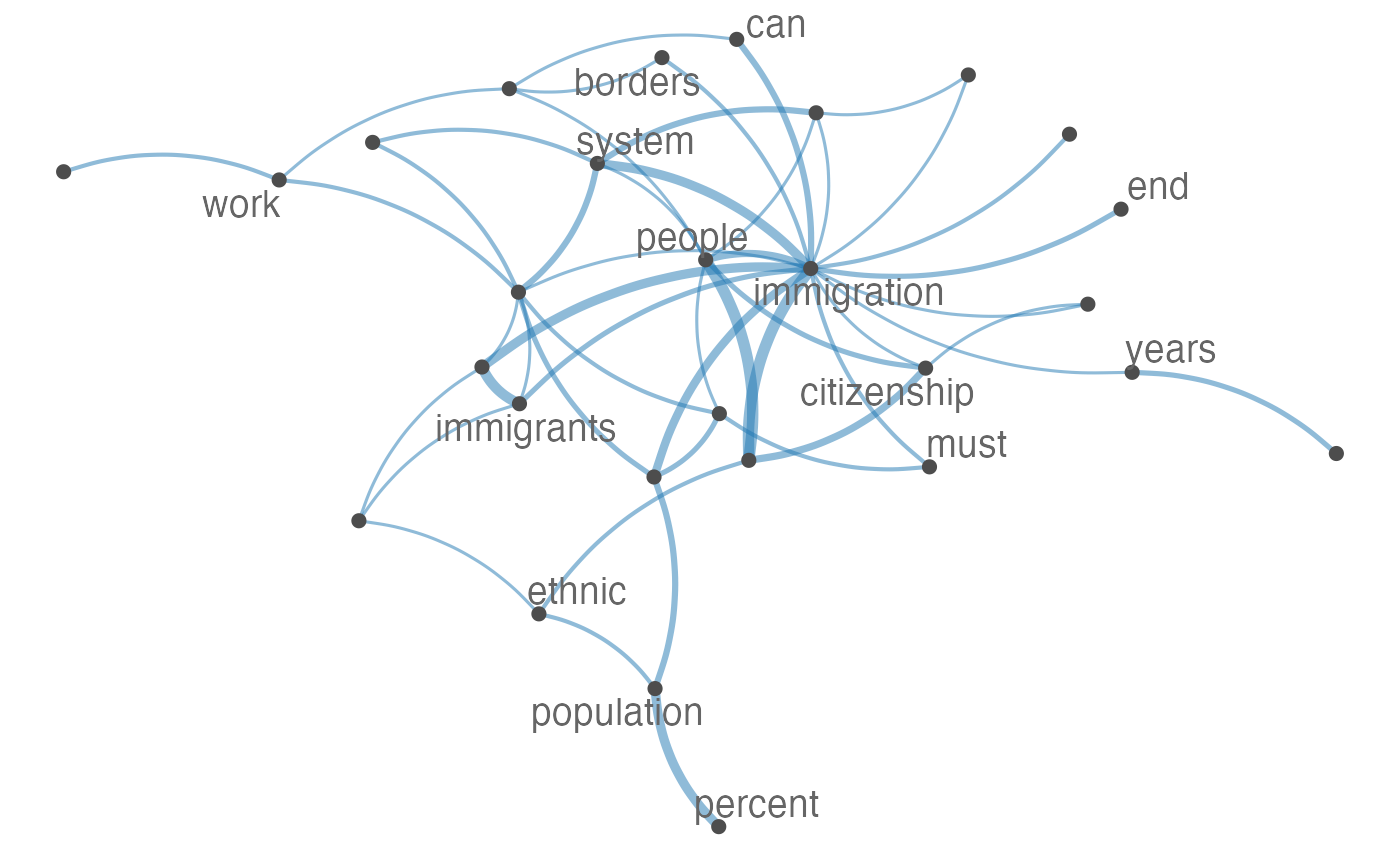fcm_select(fcmat, pattern = feat) %>%
textplot_network(vertex_labelsize = 10)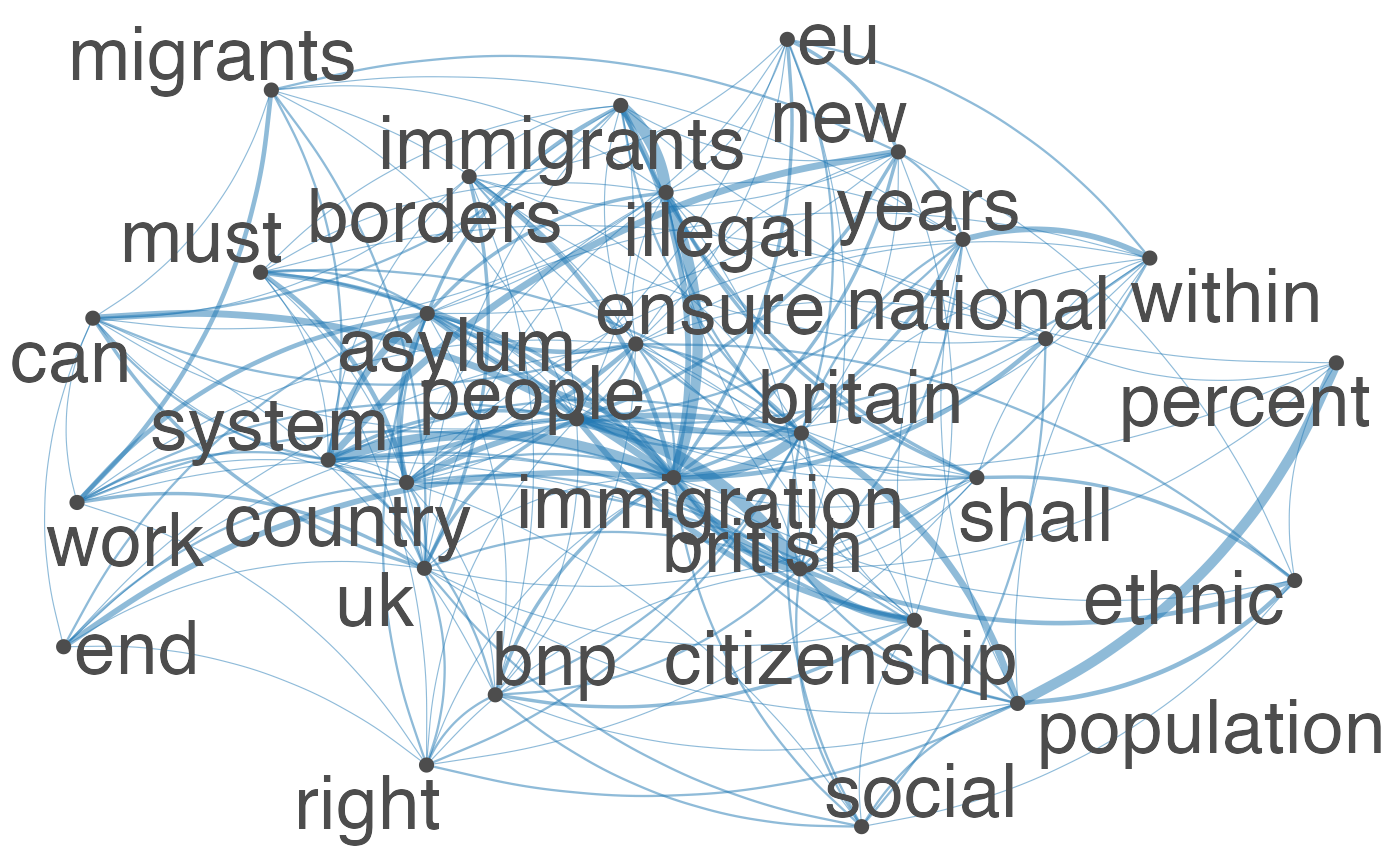fcm_30 <- fcm_select(fcmat, pattern = feat)
textplot_network(fcm_30, vertex_labelsize = rowSums(fcm_30)/min(rowSums(fcm_30)))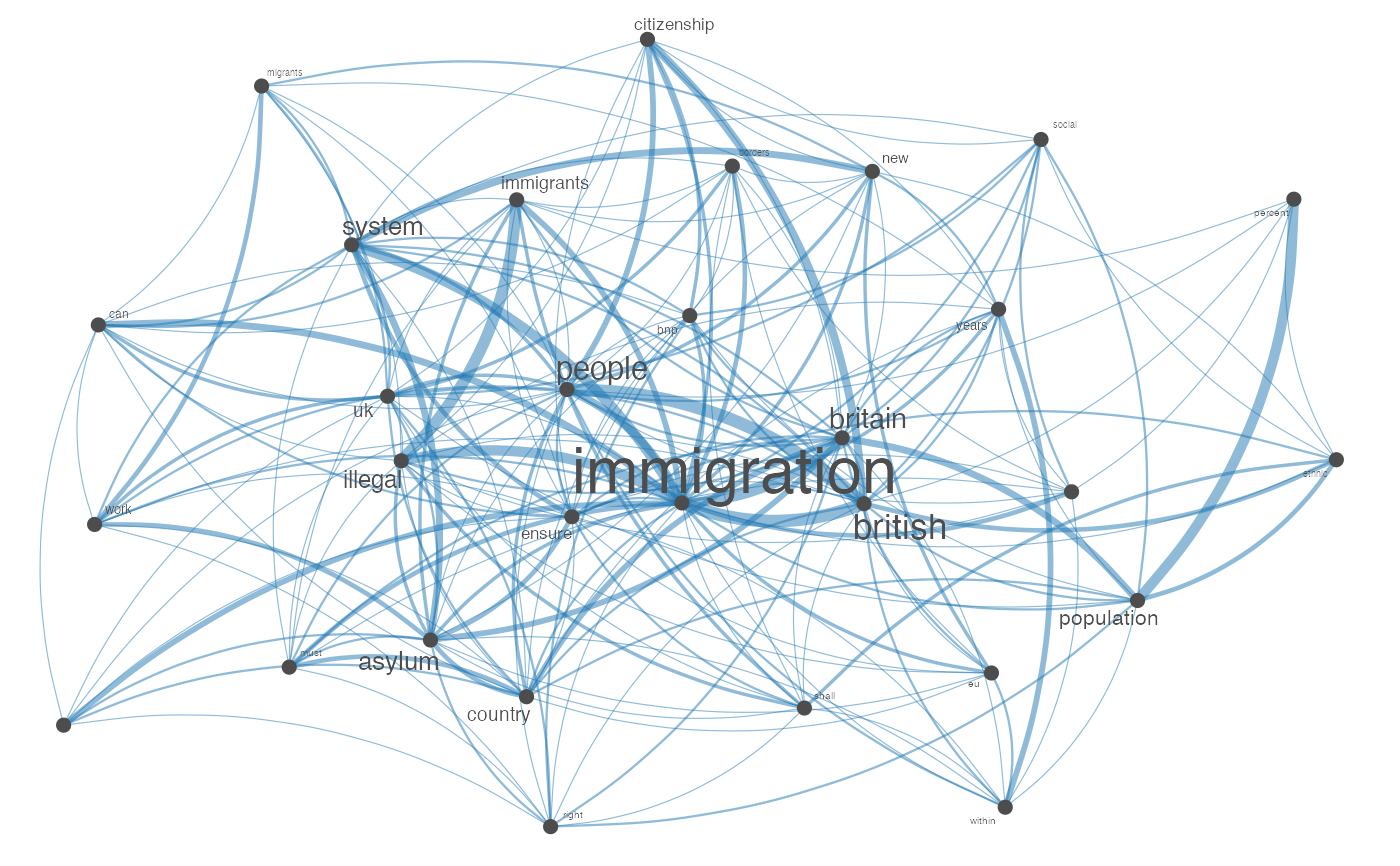# Vector inputs to vertex_labelsize can be scaled if too small / large
textplot_network(fcm_30, vertex_labelsize = 1.5 * rowSums(fcm_30)/min(rowSums(fcm_30)))# as.igraph
if (requireNamespace("igraph", quietly = TRUE)) {
txt <- c("a a a b b c", "a a c e", "a c e f g")
mat <- fcm(txt)
as.igraph(mat, min_freq = 1, omit_isolated = FALSE)
}
#> IGRAPH b8beaee DN-- 6 26 --
#> + attr: name (v/c), frequency (v/n)
#> + edges from b8beaee (vertex names):
#>   a->b a->b a->b a->b a->b a->b a->c a->c a->c a->c a->c a->c b->c b->c a->e
#>  a->e a->e c->e c->e a->f c->f e->f a->g c->g e->g f->g